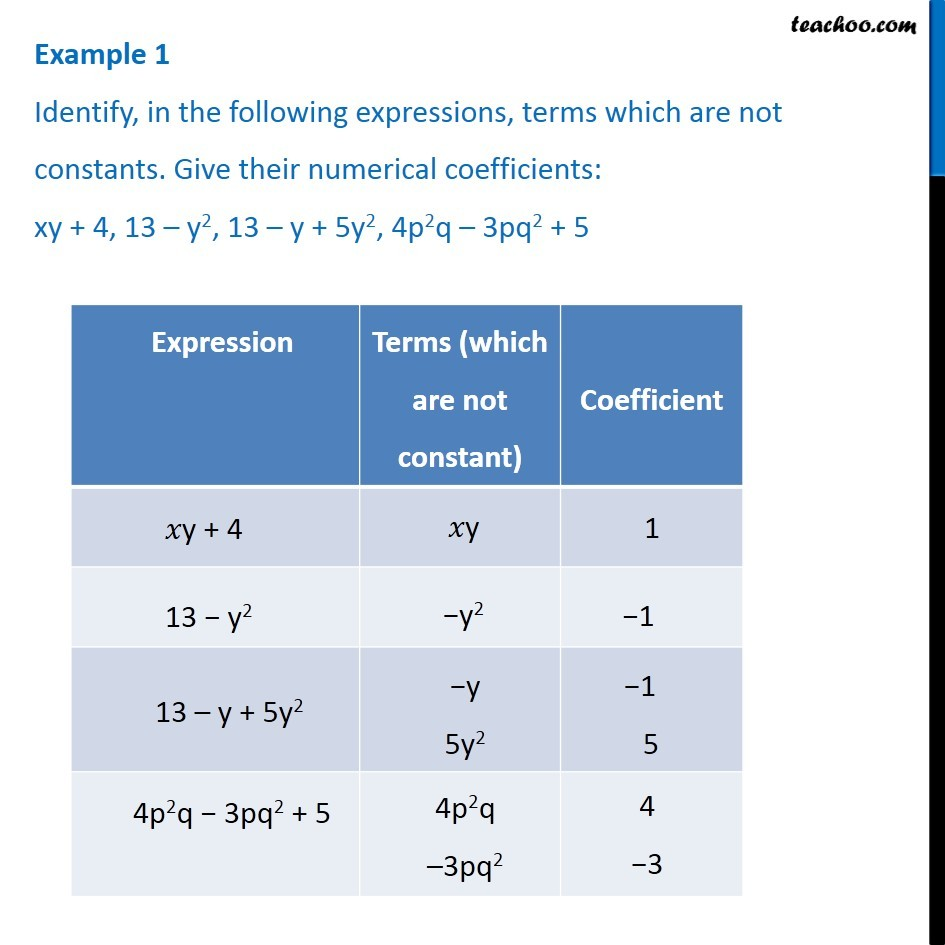1. Chapter 12 Class 7 Algebraic Expressions
2. Serial order wise
3. Examples

Transcript

Example 1 Identify, in the following expressions, terms which are not constants. Give their numerical coefficients: xy + 4, 13 – y2, 13 – y + 5y2, 4p2q – 3pq2 + 5 Expression Terms (which are not constant) Coefficient xy + 4 xy 1 13 – y2 −y2 −1 13 – y + 5y2 −y5y2 −1 5 4p2q − 3pq2 + 5 4p2q–3pq2 4 −3

Examples